1. /
2. CBSE
3. /
4. Class 06
5. /
6. Mathematics
7. /
8. NCERT Solutions for Class...

# NCERT Solutions for Class 6 Maths Exercise 13.2

NCERT solutions for Maths Symmetry## NCERT Solutions for Class 6 Maths Symmetry

###### Question 1.Find the number of lines of symmetry for each of the following shapes: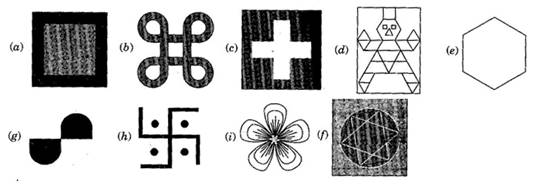(a) 4, (b) 4, (c) 4, (d) 1, (e) 6, (f) 4, (g) 0, (h) 0, (i) 3

###### Question 2. Copy the triangle in each of the following figures, on squared paper. In each case, draw the line(s) of symmetry. If any and identity the type of triangle. (Some of you may like to trace the figures and try paper-folding first!)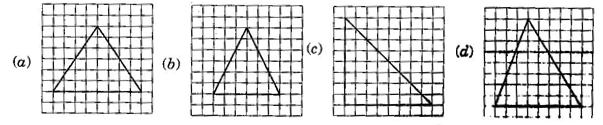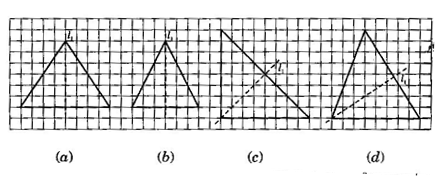(a) {tex}{l_1}{/tex} is the line of symmetry.

(b) {tex}{l_1}{/tex} is the line of symmetry.

(c) {tex}{l_1}{/tex} is the line of symmetry.

(d) No line of symmetry.

NCERT Solutions for Class 6 Maths Exercise 13.2

###### Question 3.Complete the following table:
 Shape Rough figure No. of lines of symmetry Equilateral triangle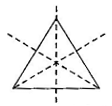3 Square Rectangle Isosceles triangle Rhombus Circle

 Shape Rough figure No. of lines of symmetry Equilateral triangle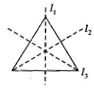3 Square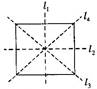4 Rectangle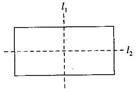2 Isosceles triangle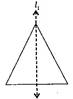1 Rhombus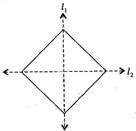2 Circle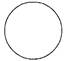Infinite

NCERT Solutions for Class 6 Maths Exercise 13.2

###### Question 4.Can you draw a triangle which has:

(a) exactly one line of symmetry?

(b) exactly two lines of symmetry?

(c) exactly three lines of symmetry?

(d) no lines of symmetry?

Sketch a rough figure in each case.

(a) Yes, Isosceles triangle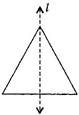(b) No such triangle cannot be formed.

(c) Yes, Equilateral triangle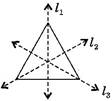(d) Yes, Scalene triangle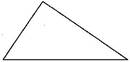###### Question 5.On a squared paper, sketch the following:

(a) A triangle with a horizontal line of symmetry but no vertical line of symmetry.

(b) A quadrilateral with both horizontal and vertical lines of symmetry.

(c) A quadrilateral with a horizontal line of symmetry but no vertical line of symmetry.

(d) A hexagon with exactly with two lines of symmetry.

(e) A hexagon with six lines of symmetry.

(Hint: It will be helpful if you first draw the lines of symmetry and then complete the figures)

(a)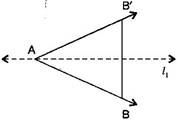(b)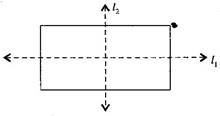(c)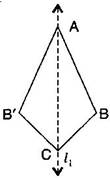(d)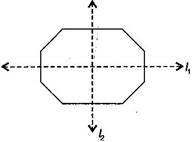(e)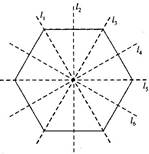NCERT Solutions for Class 6 Maths Exercise 13.2

###### Question 6.Trace each figure and draw the lines of symmetry, if any: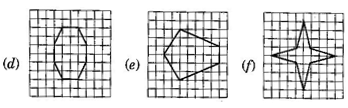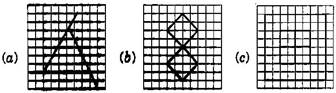(a) No line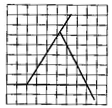(b) Two lines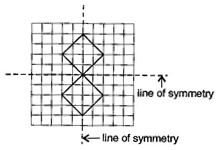(c) Four lines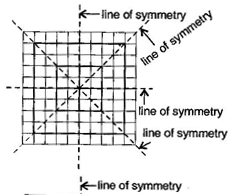(d) Two lines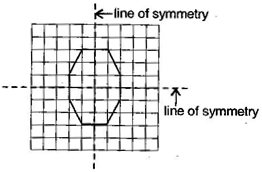(e) One line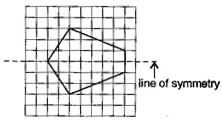(f)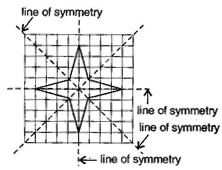###### Question 7.Consider the letters of English alphabets A to Z. List among them the letters which have:

(a) vertical lines of symmetry (like A)

(b) horizontal lines of symmetry (like B)

(c) no lines of symmetry (like Q)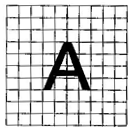Vertical lines: A, H, I, M, O, T, U, V, W, X, Y

Horizontal lines: B, C, D, E, H, I, K, O, X

No line of symmetry: F, G, J, I, N, P, Q, R, S, Z

NCERT Solutions for Class 6 Maths Exercise 13.2

###### Question 8.Given here are figures of a few folded sheets and designs drawn about the fold. In each case, draw a rough diagram of the complete figure that would be seen when the design is cut off.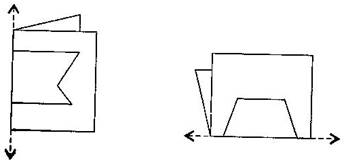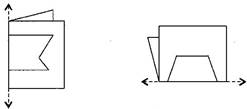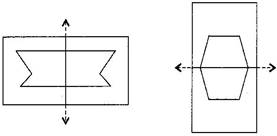## NCERT Solutions for Class 6 Maths Exercise 13.2

NCERT Solutions Class 6 Maths PDF (Download) Free from myCBSEguide app and myCBSEguide website. Ncert solution class 6 Maths includes text book solutions from Class 6 Maths Book . NCERT Solutions for CBSE Class 6 Maths have total 14 chapters. 6 Maths NCERT Solutions in PDF for free Download on our website. Ncert Maths class 6 solutions PDF and Maths ncert class 6 PDF solutions with latest modifications and as per the latest CBSE syllabus are only available in myCBSEguide.

## CBSE app for Students

To download NCERT Solutions for Class 6 Maths, Social Science Computer Science, Home Science, Hindi English, Maths Science do check myCBSEguide app or website. myCBSEguide provides sample papers with solution, test papers for chapter-wise practice, NCERT solutions, NCERT Exemplar solutions, quick revision notes for ready reference, CBSE guess papers and CBSE important question papers. Sample Paper all are made available through the best app for CBSE students and myCBSEguide website.Test Generator

Create question paper PDF and online tests with your own name & logo in minutes.myCBSEguide

Question Bank, Mock Tests, Exam Papers, NCERT Solutions, Sample Papers, Notes

### 11 thoughts on “NCERT Solutions for Class 6 Maths Exercise 13.2”

1. Thank u

2. Thank you

3. It’s a very useful thing

5. I like it very much

6. It helps me to get ready for my examination

7. i realy like this

8. Thanks for helping

9. Thanks for your help and support

10. Like this.. thanks for helping

11. Thanks to helping us